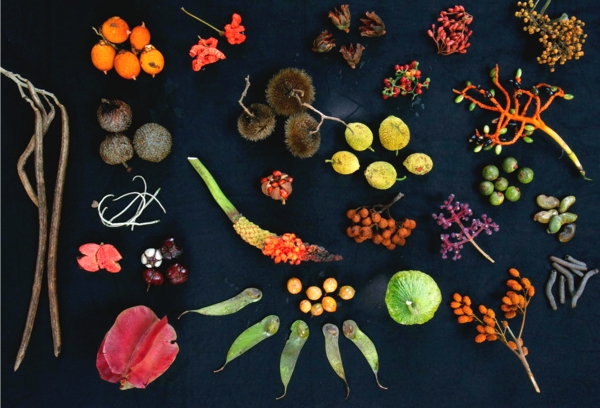## Diversity, Entropy and ThermodynamicsAs is well known, some popular measures of biodiversity are formally identical to measures of entropy developed by Shannon, Rényi and others. This fact is part of a larger analogy between thermodynamics and the mathematics of biodiversity, which we explore here. Any probability distribution can be extended to a 1-parameter family of probability distributions where the parameter has the physical meaning of 'temperature'. This allows us to introduce thermodynamic concepts such as energy, entropy, free energy and the partition function in any situation where a probability distribution is present — for example, the probability distribution describing the relative abundances of different species in an ecosystem. The Rényi entropy of this probability distribution is closely related to the change in free energy with temperature. We give one application of thermodynamic ideas to population dynamics, coming from the work of Marc Harper: as a population approaches an 'evolutionary optimum', the amount of Shannon information it has 'left to learn' is nonincreasing. This fact is closely related to the Second Law of Thermodynamics.